# Pulse Code Modulation

Pulse-code modulation or PCM is known as a digital pulse modulation technique . In fact, the pulse-code modulation is quite complex as compared to the analog pulse modulation techniques i.e. PAM, PWM and PPM, in the sense that the message signal is subjected to a great number of operations .

In PCM an anlog signal or information is converted  into a binary sequence, i.e.,’ 1’s and ‘0’s. The output of a PCM resembles a binary sequence.

The following figure shows an example of PCM output with respect to instantaneous values of a given sine wave.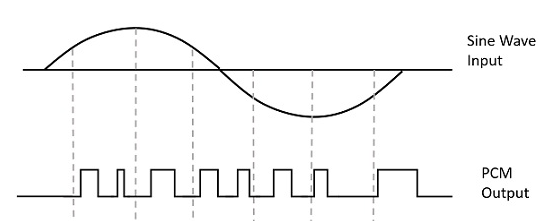PCM produces a series of numbers or digits  instead of a pulse train,. Each one of these digits,  in binary code, represent the approximate amplitude of the signal sample at that instant.

In Pulse Code Modulation, the message signal is represented by a sequence of coded pulses. This message signal is achieved by representing the signal in discrete form in both time and amplitude.

## Elements of a PCM System

Fig.1 shows the basic elements of a PCM system .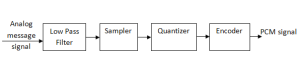(a):  PCM Transmitter(b) : Transmitter Path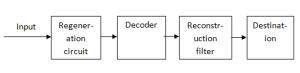Fig.1 : The basic elements of a PCM System

It consists of three main parts i.e. ,

1. Transmitter
2. Transmission path

The essential operation in the transmitter of a PCM system are :

1. Sampling
2. Quantizing
3. Encoding

As discussed earlier, sampling is the operation in which an analog (continuous-time) signal is sampled according to the sampling theorem resulting in a discrete-time signal .

The quantizing and encoding operations are usually performed in the same circuit which is known as an analog-to-digital converter (ADC) .

The essential operations in the receiver of a PCM system are :

1. Regeneration of impaired signals
2. Decoding and demodulation of the train of quantized samples

These operations are usually performed in the same circuit which is known as a digital-to-analog converter (DAC) .

Further, at intermediate points, along the transmission route from the transmitter to the receiver, regenerative repeaters are used to reconstruct (i.e. regenerate) the transmitted sequence of coded pulses in order to combat the accumulated effects of signal distortion and noise .

As discussed before, the quantization refers to the use of a finite set of amplitude levels and the selection of a level nearest to a particular sample value of the message signal as the representation for it . In fact, this operation combined with sampling, permits the use of coded pulses for representing the message signal . Thus, it is the combined use of quantizing and coding that distinguishes pulse code modulation from analog modulation techniques .

### Few Important Points :

Now, let us summarize PCM in the form of few points as under :

1. PCM is a type of pulse modulation like PAM, PWM or PPM but there is an important difference between them i.e. PAM, PWM or PPM are analog pulse modulation systems whereas PCM is a digital pulse modulation system .
2. This means that the PCM output is in the coded digital form . It is in the form of digital pulses of constant amplitude, width and position .
3. The information is transmitted in the form of code words . A PCM system consists of a PCM encoder (transmitter ) and a PCM decoder (receiver ) .
4. The essential operations in the PCM transmitter are sampling, quantizing and encoding .
5. All the operations are usually performed in the same circuit called as analog-to digital converter .
6. It should be understood that the PCM is not modulation in the conventional sense . Because in modulation, one of the characteristics of the carrier is varied in proportion with the amplitude of the modulating signal . Nothing of that sort happen in PCM .

### 1. PCM Transmitter

Fig.2 shows a practical block diagram of a PCM generator or transmitter.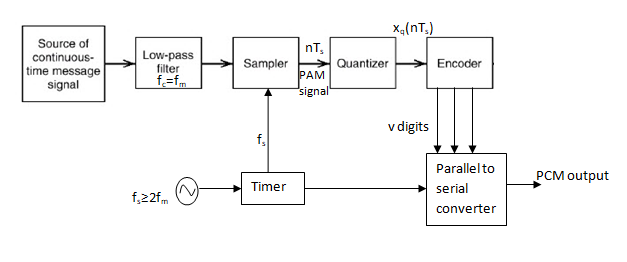Fig.2 : PCM Transmitter

In PCM transmitter , the signal x(t) is first passed through the low-pass filter of cut-off frequency fHz . This low-pass filter blocks all the frequency components above fHz.This means that now the signal x(t) is bandlimited to  fHz .

The sample and hold circuit then samples this signal at the rate of fs.

Sampling frequency f is selected sufficiently above nyquist rate to avoid aliasing i.e.,

fs  ≥ 2f

In fig.2 , the output os sample and hold circuti is denoted by x(nTs). This signal x(nTs) is discrete in time and continuous in amplitude.

A q-level quantizer compares input x(nTs) with its fixed digital levels.

It then assigns any one of the digital level to x(nTs) which results in minimum distortion or error. This error is called quantization error.

Thus output of quantizer is a digital level called xq(nTs).

Now the quantized signal level  xq(nTs) is given to binary encoder.This encoder converts input signal to ‘v’ digits binary word.This encoder is also known as digitizer.

In addition to these, there is an oscillator which generates the clocks for sample and hold circuit and parallel to serial  converter.

In PCM, sample and hold, quantizer and encoder combinely form an analog to digital converter (ADC).

### 2. PCM Transmission Path

The path between the PCM transmitter and receiver over which the PCM signal travel, is known as PCM transmission path and it is shown in Fig.3.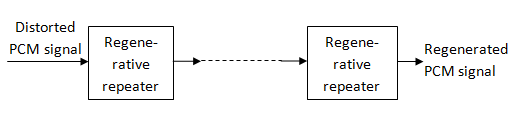Fig.3 : PCM Transmission path

The most important feature of PCM system lies in its ability to control the effects of distortion and noise when the PCM wave travels on the channel.

This is  accomplished by means of using a chain of regenerative repeaters as shown in fig.3.

Such repeaters are spaced close enough to each other on the transmission path.

The repeaters perform three basic operations such as : quantization, timing and decision making.

Hence, each repeaters actually reproduces the clean and noise free PCM signal.

This improves the performance of PCM in presence of noise.

#### Repeater

Fig.4 shows the block diagram of a repeater.

The amplitude quantizer shapes the distorted PCM wave so as to compensate for the effects of amplitude and phase distortions.

The timing circuit produces a periodic pulse train which is derived from the input PCM pulses.

This pulse train is then applied to the decision making device.

The decision making device uses this pulse train for sampling the equalized PCM pulses.

The sampling is carried out at the instants where the signal to noise ratio is maximum.

The decision device makes a decision about whether the equalized PCM wave at its input has 0 value or 1 value at the instant of sampling.

Such a decision is made by comparing equalized PCM with a reference level called decision thershold .

At the output of the decision device, we get a clean PCM signal without any noise.

Fig. 5 shows the block diagram of a PCM receiver .